# Physical Chemistry : Other Thermodynamic Principles

## Example Questions

### Example Question #1 : Other Thermodynamic Principles

If a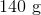copper wire gains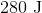of heat, what is the change in temperature of the wire?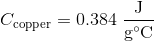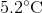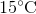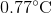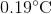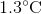Explanation:

The thermal energy an object contains is given by: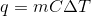Whereis the specific heat,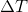is the change in temperature,is the mass, and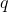is the amount of energy. We are given everything but the, so we rearrange the equation to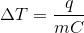Plug in known values and solve: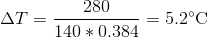### All Physical Chemistry Resources